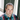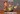# Introduction to mass spectrometry imaging data analysis

##### This post is part of our series titled "Mass spectrometry data introduction", which contains the following entries:Introduction to mass spectrometry data analysisIntroduction to mass spectrometry imaging data analysis (current post)

• Introduction
• Mass spectrometry imaging (MSI) data acquisition
• MSI data
• Example use cases
• Conclusion

• ## Introduction

##### Mass spectrometry imaging is a molecular imaging modality that can map the spatial distribution of a large number of molecules in a biological tissue section, with an unparallelled chemical specificity. MSI does not require prior tagging of molecular targets and is able to measure a large number of ions concurrently in a single experiment. Many of these advantages are due to the fact that, as the name suggests, MSI data is acquired through use of a mass spectrometer. The result data is sometimes referred to as a hyperspectral image cube, a basic illustration of which is shown in Figure 1.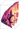## Mass spectrometry imaging (MSI) data acquisition

##### Briefly, a tissue section is cut about 8-10 micrometers thick, and mounted onto a glass ITO slide. The tissue is then covered with a chemical matrix, which assists in ionization process, and is then put in the mass spectrometer. Then, a virtual grid is laid out, and subsequently a laser is fired in each cell of this grid. This ionizes the molecules in that location, and those locally generated ions are then sent to the mass analyzer to obtain a mass spectrum is collected for that pixel. This processes is repeated for each grid cell. While other methods of ionization operate slightly differently, the overall concept of local ionization remains comparable.## MSI data

### Matrix representation of MSI data

##### When we consider high spatial resolution experiments, the data matrix is usually tall (many pixels, relatively few m/z bins), whereas high mass resolution experiments tend to give rise to wide data matrices (fewer pixels, many m/z bins). Depending on the type of data analysis, the shape of the data matrix may have a profound impact on computation time and memory requirements.
The matrix representation of MSI data mandates that all spectra are encoded using the same m/z bins (columns of D), which may require explicit realignment during data analysis. In MSI lingo, data sets in which all spectra share a common set of m/z bins are sometimes referred to as being in continuous format, based on the nomenclature used in the imzML data format.

## Example use cases

### Ion images: visualizing spatial localisation of biomolecules

##### MSI data enables us to visualize the spatial distribution of biomolecular ions with a specific mass-to-charge ratio (m/z value), by plotting the intensities for that m/z value across all pixels. In MSI terminology, the resulting visualization is called an ion image. Essentially, an ion image displays a heat map rendered using a color map of choice. Since these colors are not truly observed, they are sometimes called false color images. Figure 3 below depicts three example ion images at different m/z values.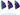##### One of the key advantages of MSI technology is that we can investigate the localisation of thousands of biomolecular ions simultaneously, whereas most other technologies are usually limited to only a handful or a couple hundred at best. Naturally, the spatial distributions of various biomolecular ions can be multiplexed onto a single image in various ways. The simplest approach is to assign color channels to different m/z values, as shown below. For more complex ways of joint visualization we refer to subsequent blog posts.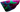### Region of interest (ROI) analysis

##### In Figure 5 below we’ve annotated two regions of interest on the ion image, shown in red and blue. A common use-case would be to compare the chemical content of those two regions.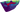##### In Figure 6, we’ve visualized the mean spectrum for each of these regions and it is immediately apparent that significant differences exist on a chemical level. In this example we’ve just computed the mean spectrum per region. In real use-cases, one might want to build classification models to distinguish these regions based on chemical information, or determine which biomolecules are differentially expressed between these regions, etc.##### Figure 6: The mean spectrum for the two regions of interest that are visualized in Figure 5.
If we would have analyzed this tissue using non-imaging MS, the first step would have been to homogenize the tissue. The resulting single mass spectrum would essentially be a convoluted mixture of the spectra of all chemically distinct regions that make up this tissue. Hence, a key strength of MSI technology is that it enables us to select MS spectra from homogenous regions within tissue, for instance within anatomical structures rather than a full organ. This opens up significant potential to identify differences on a molecular level in small regions of tissue, for which the signal would be easily overlooked using non-imaging MS.

### Detecting isotopes

##### Figure 7 shows an example of three isotopic peaks and their corresponding ion images. Based on the spectra, we can see that these peaks are roughly 1 Da apart, and thus likely to be isotopic peaks. The ion images further reveal that the spatial expressions of these peaks are near identical, which further adds to the likelihood that these are indeed isotopes. Of course the higher the mass resolution of the mass spectrometer, the more confident one can be in this assessment, and ultimately, identification would be necessary to unravel the nature of the analyte, which goes beyond the scope of this introduction.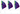## Conclusion

##### This post is part of our series titled "Mass spectrometry data introduction", which contains the following entries:Introduction to mass spectrometry data analysisIntroduction to mass spectrometry imaging data analysis (current post)